### Zero-Inflated Models

Count data that have an incidence of zeros greater than expected for the underlying probability distribution of counts can be modeled with a zero-inflated distribution. In GENMOD, the underlying distribution can be either Poisson or negative binomial. See Lambert (1992), Long (1997) and Cameron and Trivedi (1998) for more information about zero-inflated models. The population is considered to consist of two types of individuals. The first type gives Poisson or negative binomial distributed counts, which might contain zeros. The second type always gives a zero count. Letbe the underlying distribution mean and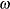be the probability of an individual being of the second type. The parameteris called here the zero-inflation probability, and is the probability of zero counts in excess of the frequency predicted by the underlying distribution. You can request that the zero inflation probability be displayed in an output data set with the PZERO keyword. The probability distribution of a zero-inflated Poisson random variable Y is given by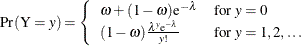and the probability distribution of a zero-inflated negative binomial random variable Y is given by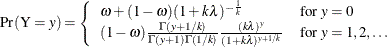where k is the negative binomial dispersion parameter.

You can model the parametersandin GENMOD with the regression models: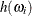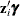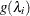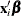where h is one of the binary link functions: logit, probit, or complementary log-log. The link function h is the logit link by default, or the link function option specified in the ZEROMODEL statement. The link function g is the log link function by default, or the link function specified in the MODEL statement, for both the Poisson and the negative binomial. The covariates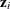for observation i are determined by the model specified in the ZEROMODEL statement, and the covariates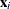are determined by the model specified in the MODEL statement. The regression parameters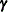andare estimated by maximum likelihood.

The mean and variance of Y for the zero-inflated Poisson are given by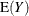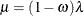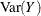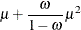and for the zero-inflated negative binomial by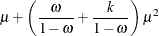You can request that the mean of Y be displayed for each observation in an output data set with the PRED keyword.